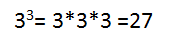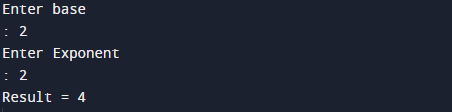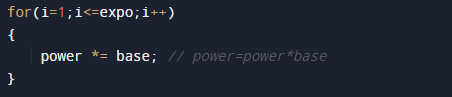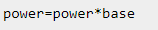# C program to find power of any number

In this tutorial you will learn about the C program to find power of any number and its application with practical example.

In this tutorial, we will learn to create a C program that will find the power of a given number  using C programming.

## Prerequisites

Before starting with this tutorial we assume that you are best aware of the following C programming topics:

• C Operators
• C  Loops.
• Basic input/output functions.

Logic behind to find power of any number without using pow() function in C programming.

## Example

### Input:

take  value of  base : 3
take  value of  exponent : 3

### Output:

Input base is : 3
Input exponent : 3## Program to find power of any number.

So power of any number basen given as base*base*…..*base (n-times). Here base is called base and n is called the exponent.Here we will write a C program to find the power of a given number.

## OutputNote: The above program will works only if the exponent is a positive integer(number).

In the above program, we have first declared and initialized a set variables required in the program.

• base = it hold the value of base
• expo = it will hold the power
• power= it will hold the result.

Let see the step by step descriptive logic.

Step 1. Input base and exponents from user. and put  it in two variables  base and expo.

Step 2. Initialize another variable that will store power power=1.

Step 3.within the loop value of i start from 1 to expo, increment loop  by 1 in each iteration. The structure  look likeStep 4.For every iteration the value of  power  multiply with base.Step 5.Finally we get the answer in Power.In this tutorial we understand how to write a program without using Power Function In C to calculate power of a number.

In this tutorial we have learn about the C program to find power of any number and its application with practical example. I hope you will like this tutorial.